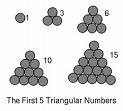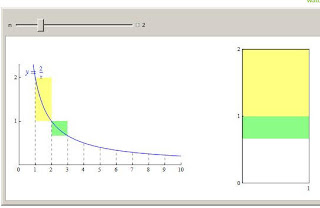## Thursday 10 September 2009

### Limits as x --> InfinityFirst week of calculus, and the talk is all about limits and infinity...

They say that one of Leibniz's earliest explorations into limits and infinite series was in finding the sum of the reciprocals of the triangular numbers, or $\sum_{1}^{\infty}\frac{2}{(n)(n+1)}\$

The triangular numbers are the numbers that can be made by adding the counting integers

1, 1+2=3, 1+2+3=6, etc.. they also appear as the third number in on each row of Pascal's arithmetic triangle, so they are the combination of n things taken two at a time. The nth triangular number then, is $\dbinom{n}{2}$ = $\frac{(n)(n+1)}{2}$

The sequence of reciprocals would then $\frac{1}{1}+\frac{1}{3}+\frac{1}{6}+...+\frac{2}{(n)(n+1)}$

But if we write them out using the $\frac{2}{(n)(n+1)}$= $\frac{2}{n}}$ - $\frac{2}{n+1}$ model for each term, then something interesting happens...

writing 1 as $\frac{2}{1}-\frac{2}{2}$ may seem silly at first, but if you follow that with 1/3 = $\frac{2}{2}-\frac{2}{3}$ you realize that when you add these together, the alternating terms eliminate each other and the sum of the first two terms is just 2/1 - 2/3 or 1 1/3. Ok, the worlds slowest way to add one to one-third.. but now lets add the next few values 2/1-2/2 + 2/2-2/3+2/3-2/4+2/4-2/5 + 2/5 - 2/6 we see that all the underlined stuff disappears and we are left with the first term, 2, minus the last term, which is 2/(n+1) for whatever term number we are at.. so the sum of the first five terms, 1+1/3+1/6+1/10+1/15 = 2-2/6 or 1 2/3.

and if we wanted the first 20 terms we could just do 2 - 2/21 or 1 19/21. and if we added them up forever???? well what happens to 2/(n+1) as n gets bigger and bigger??? ....so the infinite sum will just approach a limit of two.

After I did this in class recently, I found this nice graphic demonstration at the wolfram demonstrations page;...a proof without words."This problem was historically significant as it served as in inspiration for Leibniz to explore many more infinite series. Since he successfully solved this problem, he concluded that a sum could be found of almost any infinite series. (Boyer, 446-447)" ( Worcester Polytechnic Institute )